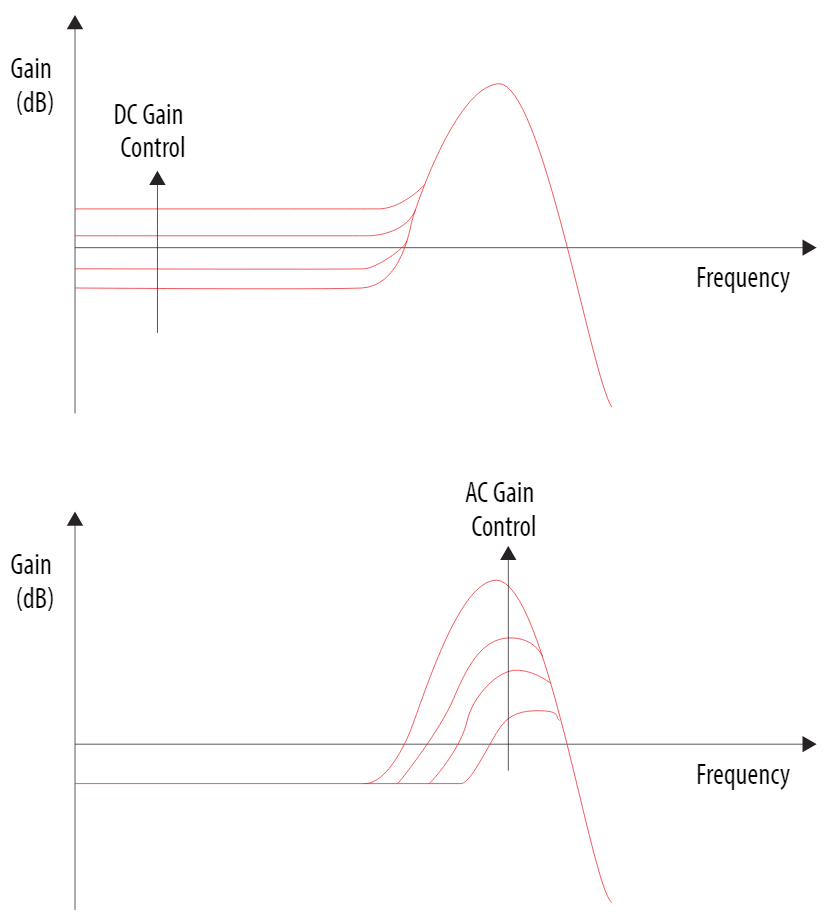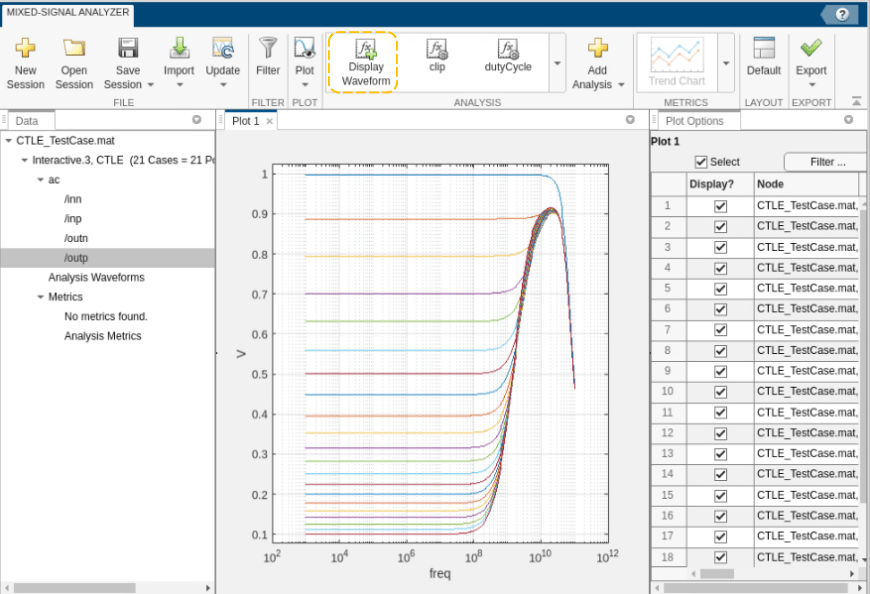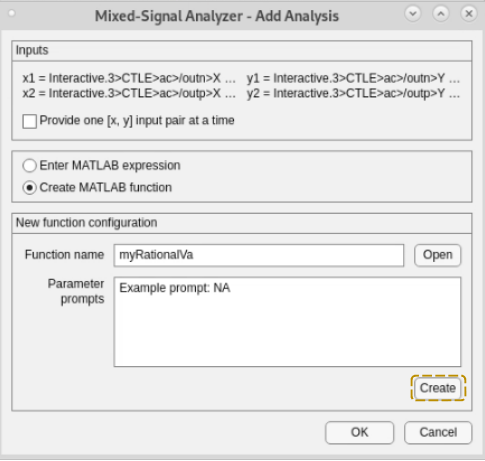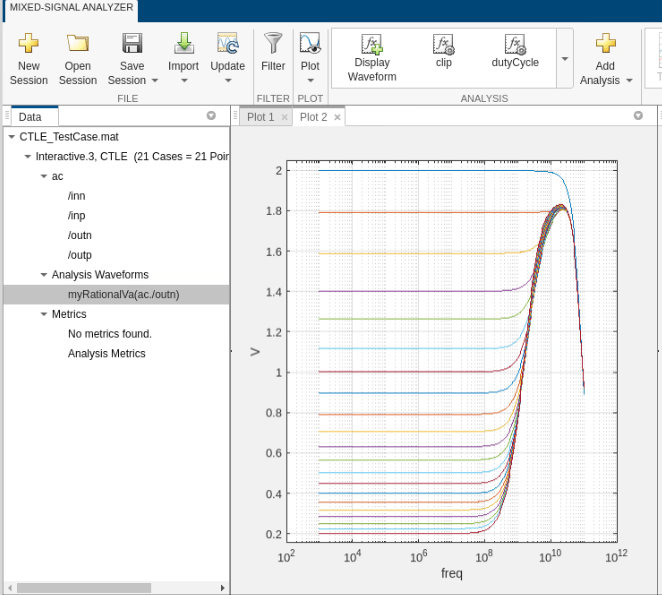# Generate VerilogA Model of CTLE Using Custom Function in MSA

This example shows you how to write custom analysis function in Mixed-Signal Analyzer (MSA) app to fit the transfer function of a CTLE circuit to a rational function and generate a VerilogA model that can be simulated in Cadence.

### CTLE

Continuous Time Linear Equalizer (CTLE) is used in Serializer/Deserializer (SerDes) systems for signal reconstruction. It applies a linear peaking filter to equalize the signal and reduce distortions resulting from lossy channels.Using the notations used in the typical CTLE circuit provided above we can define its transfer function as shown below:

`$H=\frac{outp-outn}{inp-inn}$`

The equalization circuits compensate for low-pass characteristics of the physical medium by amplifying high-frequency components of the incoming signal. The CTLE can support both DC and AC gain as shown in the plot below . DC gain control is varied in the CTLE circuit in this example by varying Rstep.### Custom Analysis in MSA

Before using MSA app, one needs to generate CTLE simulation data using Cadence Virtuoso. CTLE simulation in Cadence is performed using different gain settings and saving input and output waveforms for each setting. You can extract the cadence simulation data into a mat file for further analysis in MSA using the function adeinfo2msa(). You can provide name-value pairs as arguments to this function. More information about using this function can be found at <msblks_ref#mw_95f326b1-aad1-433d-9d4e-f8b704a29fba>.

The CTLE simulation data has been provided in a mat-file named ‘CTLE_TestCase.mat’. You can import the simulation data in MSA using the command below:

`mixedSignalAnalyzer('CTLE_TestCase.mat')`

The function opens MSA app and displays the list of waveform data brought over from Cadence in the data panel of the app. Next, you can select any signal name and click on “Display Waveform” button in the toolstrip to visualize the signal. For example, in the image below waveforms related to 21-point sweeps for signal ‘outp’ has been displayed in the app.To add a custom analysis function to fit the CTLE transfer function to a rational function, select the signals ‘/outp’ and ‘/outn’ and then click on ‘Add Analysis’ button in the toolstrip. This will pop-up an interface to perform your analysis. You can select ‘Create MATLAB function’ radio button and click on ‘Create’ to get MATLAB editor window to code your algorithm.You can modify the code template provided by the custom analysis tool to add your own code after the comment ‘Begin custom code’. The algorithm for the custom function to perform rational fit is shown below:

1. The arguments to the function are the waveforms from AC analysis. The differential output is given by (y2- y1). Here y2 = outp and y1 = outn. x1 is the frequency vector. The differential input to CTLE has a unity magnitude.

2. Perform rational fitting of the frequency domain response and obtain the response Yout

3. Export the obtained rational function to a Veriloga file for behavioral model simulation in Cadence 

Use the code shown below for implementing the above algorithm, i.e., for fitting a rational function to the CTLE output and generating a VerilogA model.

```function [Xout, Yout, NameValuePairs] = myRationalVa(x1, x2, y1, y2, dialogAnswers) %MYRATIONAL Summary of this function goes here % Detailed explanation goes here % % Inputs: % x1, ..., xN x-coordinate vectors for waveforms 1 through N % y1, ..., yN y-coordinate vectors for waveforms 1 through N % dialogAnswers struct containing responses to the parameter % dialog box. % Outputs: % Xout x-coordinate vector for output waveform % Yout y-coordinate vector for output waveform % NameValuePairs cell array. Even entries are names (text) and % odd entries are values (any type). By default, % the following names have special meanings: % xUnit 'Time' | 'Freq' units for Xout % yUnit 'Time' | 'Freq' units for Yout % xScale 'Linear' | 'Log' scaling for Xout % yScale 'Linear' | 'Log' scaling for Yout % xLabel Xout axis label (text) % yLabel Yout axis label (text) % function Name of the completed analysis % All other name-value pairs are interperted as % analysis metrics. % Comments following dialogAnswers are used as prompts for the input dialog % box. Modifying these comments will change the prompts. arguments x1 {mustBeNumeric, mustBeVector}; x2 {mustBeNumeric, mustBeVector}; y1 {mustBeNumeric, mustBeVector}; y2 {mustBeNumeric, mustBeVector}; dialogAnswers.Prompt1 {mustBeNonzeroLengthText} = '0'; % Example prompt: NA end %Initialize returned values Xout = x1; % Yout = y1; NameValuePairs = {}; % Begin custom code. persistent n; if isempty(n) n = 1; end rationalObject = rational(x1,y2-y1); Yout = freqresp(rationalObject,x1); writeva(rationalObject,['file' num2str(n)]); n = n+1; semilogx(x1, 20*log10(abs(Yout))); hold on; semilogx(x1, 20*log10(abs(y2-y1))); end```

After editing the function, save the file and click on ‘OK’ on the custom analysis prompt. The waveform ‘myRationalVa’ should appear under ‘Analysis Waveforms’. To display the waveforms first click on ‘Plot’ to add a new tabbed plot window. Now, select ‘myRationalVa’ and click on ‘Display Waveform’.